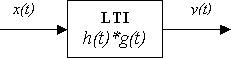Linear Time-Invariant Systems and Convolution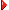Welcome! ` ` An Interactive Lecture by Wilson J. Rugh ` ` aboutSignals and Systems
A continuous-time signal is a function of time, for example written x(t), that we assume is real-valued and defined for all t,
-¥ < t < ¥ .

A continuous-time system accepts an input signal, x(t), and produces an output signal, y(t). A system is often represented as an operator "S" in the form

y(t) = S [x(t)]LTI Systems
A linear continuous-time system obeys the following property: For any two input signals x1(t), x2(t), and any real constant a, the system responses satisfy

S [ x1(t) + x2(t)] = S [x1(t)] + S [x2(t)]

and

S [ a x1(t) ] = a S [x1(t) ]

A time-invariant system obeys the following time-shift invariance property: If the response to the input signal x(t) is

y(t) = S [x(t)]

then for any real constant T,

y(t - T) = S [x(t - T)]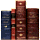Examples of LTI Systems
Simple examples of linear, time-invariant (LTI) systems include the constant-gain system,

y(t) = 3 x(t)

and linear combinations of various time-shifts of the input signal, for example

y(t) = 3x(t) - 2 x(t - 4) + 5 x(t + 6)Convolution Representation
A system that behaves according to the convolution integral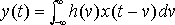where h(t) is a specified signal, is a linear time-invariant system. Essentially all LTI systems can be represented by such an expression for suitable choice of h(t).In the convolution expression, the integrand involves the product of two signals, both functions of the integration variable, v. One of the signals, x(t - v), involves a transformation of the integration variable and introduces t as a parameter. To explore this transformation, select or draw with the mouse a signal x(v) in the first window below. Then click on the v axis in the second window to set a value of t and the signal x(t - v) will appear. The value of t can be changed by dragging it with the mouse.Flip and Shift

You need a Java-compatible browser to see this demo.

Given the signals h(t) and x(t), mathematically evaluating y(t) from the convolution integral is straightforward in principle. But even for simple signals h(t) and x(t) the bookkeeping can be complicated. To explore graphical evaluation of y(t), select or draw the signals x(t) and h(t) in their respective windows below, and then click on the first v-axis to set the value of t. Upon dragging t with the mouse, the individual signals that are multiplied to form the integrand, the integrand, and the output signal are displayed in their respective windows.Joy of Convolution

You need a Java-compatible browser to see the demo.Impulse Response

The signal h(t) that describes the behavior of the LTI system is called the impulse response of the system, because it is the output of the system when the input signal is the unit-impulse, x(t) = d (t). We also permit impulses in h(t) in order to represent LTI systems that include constant-gain examples of the type shown above.LTI System Properties

An LTI system is called causal if the output signal value at any time t depends only on input signal values for times less than t. It is easy to see from the convolution integral that if h(t) = 0 for t < 0, then the system is causal.

An LTI system is called memoryless if the output signal value at any time t depends only on the input signal value at that same time. Again from the convolution integral, if h(t) = 0 for all nonzero values of t, the system is memoryless. Of course in this situation the integrand is zero for all nonzero values of v, and obviously the output signal will be identically zero unless h(t) has an impulse at the origin. If h(t) = a d (t), for some real constant a, then the system is memoryless. In other words, a constant-gain system is a memoryless system .

An LTI system is called stable if every bounded input signal produces a bounded output signal. A signal x(t) is bounded if there is a finite constant b such that the absolute value of the signal satisfies | x(t) | < b, for all t. The following, short calculation shows that if the impulse response of an LTI system is absolutely integrable,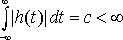then the system is stable. Assuming that there exists such b, c, and using the fact that the absolute value of an integral is no greater than the integral of the absolute value, for any t we obtain the bound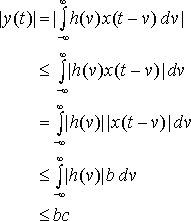Properties of *

From a mathematical viewpoint, convolution can be viewed as a special operation on two signals that produces a third signal, and the notation

y(t) = h(t)* x(t)

is used. Basic mathematical properties of this operation to be explored are commutativity, associativity, and distributivity.That the operation * is commutative, that is,
h(t)* x(t) = x(t)* h(t)

is believable from experimentation with graphical convolution. The proof involves performing a change of integration variable on the underlying integral formula. Begin with h(t)* x(t),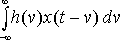and let w = t Ł v. Substituting gives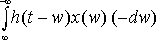an expression that rearranges to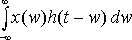Of course this is x(t)* h(t), and thus * commutes.

In showing that a system described by convolution is linear, the property that convolution distributes over addition of signals is confirmed:

h(t)* [x(t) + z(t)] = h(t)* x(t) + h(t)* z(t)

It is more tedious to show that convolution is associative,

h(t)* [x(t)* z(t)] = [ h(t)* x(t) ]* z(t)

from the integral formula, and the extra work is simply in the book-keeping required to write out the two expressions and relate them by a change of integration variable.Algebraic Properties of *

Commutativity implies that the response of a system with impulse response h(t) to the input signal x(t) is identical to the response of a system with impulse response x(t) to the input h(t).

Associativity implies that in a cascade of LTI systems, where the output of one system is the input to the next, the ordering of the systems does not affect the overall input-output behavior. In terms of diagrams, where it is natural and convenient to display an LTI system in the form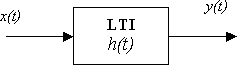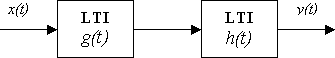has the same behavior from input signal to output signal as the system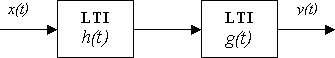and, by associativity, either of these cascade systems can be represented as